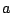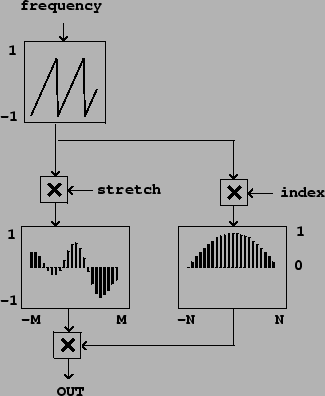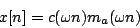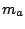Next: Pulse trains Up: Designer spectra Previous: Designer spectra   Contents   Index

# Carrier/modulator model

Earlier we saw how to use ring modulation to modify the spectrum of a periodic signal, placing spectral peaks in specified locations (see Figure 5.4, Page). To do so we need to be able to generate periodic signals whose spectra have maxima at DC and fall off monotonically with increasing frequency. If we can make a signal with a formant at frequency zero--and no other formants besides that one--we can use ring modulation to displace the formant to any desired harmonic. If we use waveshaping to generate the initial formant, the ring modulation product will be of the formwhere(the carrier frequency) is set to the formant center frequency andis a signal with fundamental frequency determined by, produced using a waveshaping functionand index. This second term is the signal we wish to give a formant at DC with a controllable bandwidth. A block diagram for synthesizing this signal is shown in Figure 6.2.Much earlier in Section 2.4 we introduced the technique of timbre stretching, as part of the discussion of wavetable synthesis. This technique, which is capable of generating complex, variable timbres, can be fit into the same framework. The enveloped wavetable output for one cycle is:where, the phase, satisfies. Hereis a function stored in a wavetable,is a windowing function, andandare the wavetable stretching and a modulation index for the windowing function. Figure 6.3 shows how to realize this in block diagram form. Comparing this to Figure 2.7, we see that the only significant new feature is the addition of the index.

In this setup, as in the previous one, the first term specifies the placement of energy in the spectrum--in this case, with the parameteracting to stretch out the wavetable spectrum. This is the role that was previously carried out by the choice of ring modulation carrier frequency.Both of these (ring modulated waveshaping and stretched wavetable synthesis) can be considered as particular cases of a more general approach which is to compute functions of the form,whereis a periodic function describing the carrier signal, andis a periodic modulator function which depends on an index. The modulation functions we're interested in will usually take the form of pulse trains, and the indexwill control the width of the pulse; higher values ofwill give narrower pulses. In the wavetable case, the modulation function must reach zero at phase wraparound points to suppress any discontinuities in the carrier function when the phase wraps around. The carrier signal will give rise to a single spectral peak (a formant) in the ring modulated waveshaping case; for wavetables, it may have a more complicated spectrum.

In the next section we will further develop the two forms of modulating signal we've introduced here, and in the following one we'll look more closely at the carrier signal.Next: Pulse trains Up: Designer spectra Previous: Designer spectra   Contents   Index
Miller Puckette 2006-12-30